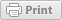# What is the Rule of 72? How is it used in investing?

Personal Finance February 28, 2013The Rule of 72 is useful for understanding compound interest because it shows the approximate time or interest rate required to double a sum of money (e.g., \$2,500 to \$5,000).

To apply the Rule of 72 to your own personal investments, you need to know or assume their expected rate of return. For example, you may own a bond that you know will regularly pay 6% interest until maturity or assume that the average annual return on your stocks is 8%.

Simply divide 72 by the known or assumed interest rate. The result is the amount of time that it will take to double a sum of money. For example, at a 6% interest rate, money will double in approximately 12 years (72/6). At 8% interest, the doubling period is shorter, 9 years (72/8).

You can also calculate the Rule of 72 with the doubling time as the assumed variable. Simply divide that number into 72 to calculate the interest rate required to double your money within that time frame. For example, if you want to double your money in 10 years, you’ll need to earn about a 7% average annual return (72 divided by 10 = 7.2).

We would like your feedback on this Personal Finance Frequently Asked Question.

### Welcome

This is where you can find research-based information from America's land-grant universities enabled by eXtension.orgThis work is supported by the USDA National Institute of Food and Agriculture, New Technologies for Ag Extension project.